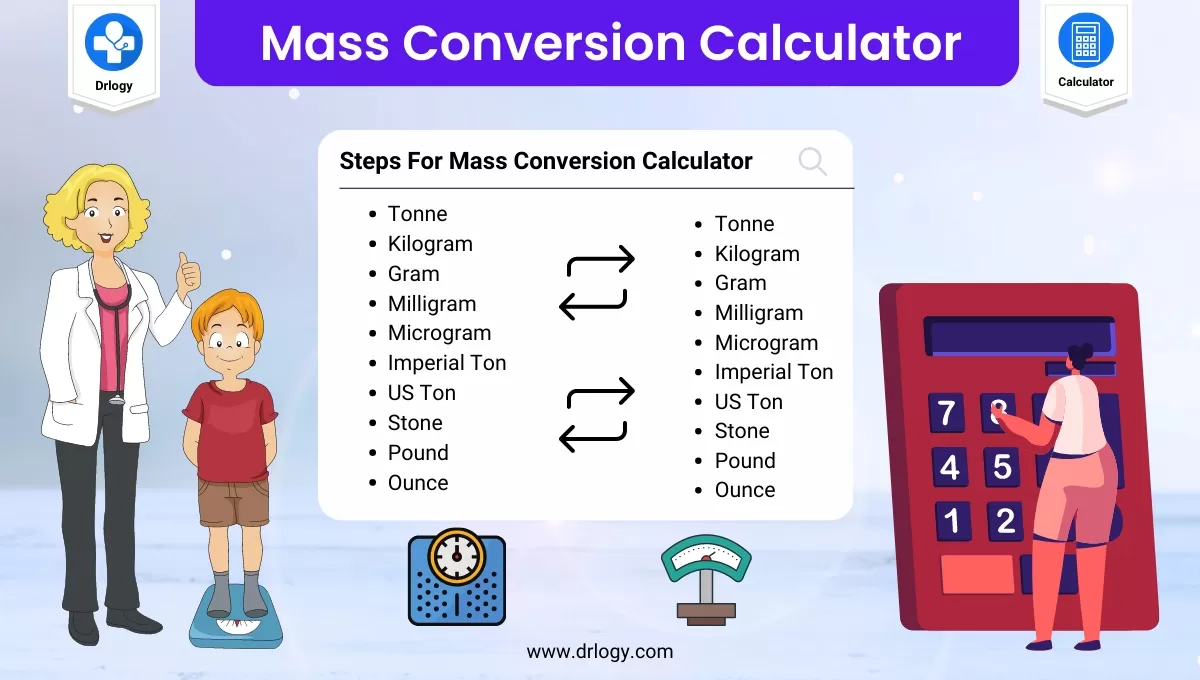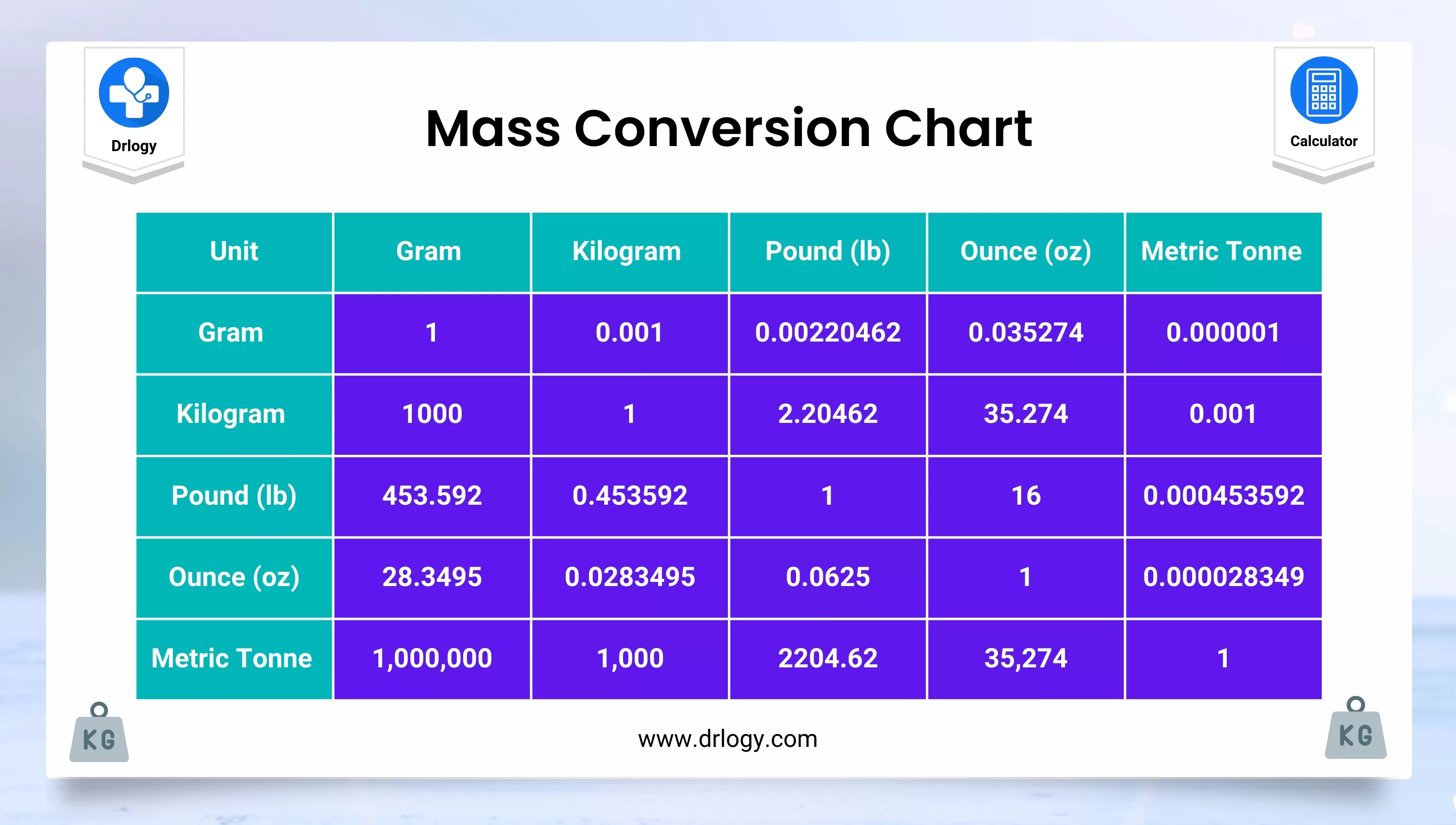• Health
• Pet
• Unit Conversion
• Medical# Mass Conversion Calculator With Chart And Table

## Mass Conversion Calculator

Result : =
keyboard_arrow_down
=
keyboard_arrow_down

## Mass Conversion Calculator Overview

• Mass Conversion Calculator is designed to help you convert between different units of mass, such as grams, kilograms, pounds, and ounces.
• To use the calculator, simply enter the starting mass value and the unit of measurement for that value, then select the unit of measurement you want to convert to.
• The calculator will then use the appropriate conversion factor to calculate the converted mass value for you.
• Keep in mind that there may be slight variations in conversion factors depending on the specific units involved, so double-check your work to ensure accuracy.

## Steps For Mass Conversion Calculator

Here are the general steps you can follow to use a mass conversion calculator:

1. Determine the units of measurement for the starting and ending mass values. For example, if you want to convert 500 grams to ounces, the starting unit is grams and the ending unit is ounces.
2. Look up the conversion factor between the two units. You can do this by searching online or consulting a conversion chart.
3. Multiply the starting mass value by the conversion factor. For example, if the conversion factor between grams and ounces is 0.035274, you would multiply 500 grams by 0.035274 to get 17.637 ounces.
4. Round the answer to an appropriate number of significant figures. For example, if the original mass value was only known to two significant figures, you should round the converted value to two significant figures as well.
5. Double-check your work to ensure that you've used the correct units and conversion factor and that your answer makes sense.

## Mass Conversion Units

Here are some common units of mass and their abbreviations:

• Milligram (mg)
• Gram (g)
• Kilogram (kg)
• Metric tonne (t)
• Pound (lb)
• Ounce (oz)

Note that there are many other units of mass, especially in different systems of measurement, but these are some of the most commonly used ones. When converting between different units of mass, it's important to use the correct conversion factor to ensure accurate results.

## Mass Conversion Chart

Here's a chart with some common mass conversions:

From To Conversion Factor
Milligrams (mg) Grams (g) 0.001
Grams (g) Kilograms (kg) 0.001
Kilograms (kg) Pounds (lb) 2.20462
Pounds (lb) Ounces (oz) 16
Ounces (oz) Grams (g) 28.3495
Grams (g) Ounces (oz) 0.035274
Metric Tons (t) Kilograms (kg) 1000
Kilograms (kg) Metric Tons (t) 0.001
Short Tons (ton) Pounds (lb) 2000
Pounds (lb) Short Tons (ton) 0.0005

Note that there are many other possible mass conversions, depending on the specific units involved.## Mass Conversion Formula

Here are some common formulas for mass conversion:

### Grams to kilograms

• divide the number of grams by 1000
• kilograms = grams / 1000

### Kilograms to grams

• multiply the number of kilograms by 1000
• grams = kilograms x 1000

### Pounds to ounces

• multiply the number of pounds by 16
• ounces = pounds x 16

### Ounces to pounds

• divide the number of ounces by 16
• pounds = ounces / 16

### Kilograms to pounds

• multiply the number of kilograms by 2.20462
• pounds = kilograms x 2.20462

### Pounds to kilograms

• Divide the number of pounds by 2.20462
• kilograms = pounds / 2.20462
• Note that there may be other formulas depending on the specific units involved, but these are some of the most commonly used ones.
• When converting between units, it's important to ensure that you use the correct conversion factor or formula to obtain accurate results.

## Mass Conversion Table

Starting Multiplier Ending
Ounce (oz) 0.0625 Pound (lb)
Ounce (oz) 2.835e+7 Microgram (microg)
Ounce (oz) 28,349.5 Milligram (mg)
Ounce (oz) 28.349 Gram (g)
Ounce (oz) 0.028349 Kilogram (kg)
Pound (lb) 16 Ounce (oz)
Pound (lb) 4.536e+8 Microgram (microg)
Pound (lb) 453,592 Milligram (mg)
Pound (lb) 453.592 Gram (g)
Pound (lb) 0.453592 Kilogram (kg)
Microgram (microg) 3.5274e-8 Ounce (oz)
Microgram (microg) 2.2046e-9 Pound (lb)
Microgram (microg) 0.001 Milligram (mg)
Microgram (microg) 1e-6 Gram (g)
Microgram (microg) 1e-9 Kilogram (kg)
Milligram (mg) 3.5274e-5 Ounce (oz)
Milligram (mg) 2.2046e-6 Pound (lb)
Milligram (mg) 1000 Microgram (microg)
Milligram (mg) 0.001 Gram (g)
Milligram (mg) 1e-6 Kilogram (kg)
Gram (g) 0.035274 Ounce (oz)
Gram (g) 0.00220462 Pound (lb)
Gram (g) 1e+6 Microgram (microg)
Gram (g) 1000 Milligram (mg)
Gram (g) 0.001 Kilogram (kg)
Kilogram (kg) 35.274 Ounce (oz)
Kilogram (kg) 2.20462 Pound (lb)
Kilogram (kg) 1e+9 Microgram (microg)
Kilogram (kg) 1e+6 Milligram (mg)
Kilogram (kg) 1,000 Gram (g)

## Mass Conversion Calculator Benefits

Here are some benefits of using a mass conversion calculator:

1. Accuracy: Mass conversion calculations can be complex, and using a calculator helps to eliminate errors that can occur when performing calculations manually.
2. Efficiency: A mass conversion calculator allows you to quickly and easily convert between different units of mass without having to perform calculations manually or consult conversion charts.
3. Convenience: With a mass conversion calculator, you can convert between mass units wherever you are, as long as you have access to a device with an internet connection.
4. Saves time: Using a mass conversion calculator saves time that would otherwise be spent on manual calculations or searching for conversion factors.
5. Accessibility: A mass conversion calculator can be used by anyone, regardless of their mathematical ability or familiarity with mass conversions.

Summary

Overall, a mass conversion calculator can save time and increase accuracy when converting between different mass units, making it a valuable tool for anyone who needs to perform mass conversions on a regular basis. Check more unit conversion calculators like this to solve your daily problems on Drlogy Calculator to get exact solution.

Reference

• Conservation of mass - Wikipedia .
• Weight and Mass Conversion - FREE Unit Converter .

favorite_border 8474 Likes

## Mass Conversion Calculator FAQ

### What is mass conversion?

• Mass conversion is the process of converting a value of mass from one unit of measurement to another.
• Mass is a measure of the amount of matter in an object and is typically measured in units such as grams, kilograms, pounds, and ounces.
• Mass conversion is often necessary when working with different systems of measurement or when comparing values measured in different units.
• To convert mass from one unit to another, you need to use the appropriate conversion factor or formula that relates the two units of measurement.
• This can be done manually using conversion tables or formulas, or by using a mass conversion calculator.

### How do you convert mass to kg?

• To convert mass to kilograms, you need to divide the mass value by 1000. This is because there are 1000 grams in one kilogram. Here's the formula for converting mass to kilograms:

Mass in kilograms = Mass in grams / 1000

• For example, if you have a mass value of 5000 grams, you can convert it to kilograms using the following calculation:

Mass in kilograms = 5000 / 1000 = 5 kg

• Therefore, 5000 grams is equivalent to 5 kilograms.

### How do you convert 100g to 1kg?

To convert 100g to 1kg, you need to divide the mass value by 1000. This is because there are 1000 grams in one kilogram.

Here's how to do it:

1. Convert the starting mass value of 100g to kilograms by dividing it by 1000:

100g ÷ 1000 = 0.1 kg

1. Compare the resulting value of 0.1 kg to 1 kg.

Since 0.1 kg is smaller than 1 kg, you can say that 100g is equivalent to 0.1 kg.

Therefore, 100g is equal to 0.1 kg.

View More Health

## Medical Calculator

View More Medical

View More Pet

## Unit Conversion Calculator

View More Unit Conversion

## Financial Calculator

View More Financial
Drlogy®
The Power To Health